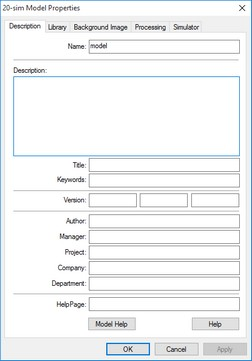﻿ 20-sim webhelp > Editor > Using Models > Model Properties

# Model Properties

The properties of 20-sim models are shown in the Model Properties dialog. You can open the Model Properties in various ways.

 • Select the proper model in the Model Browser and then click Properties from the Right Mouse menu.
 • Select the model in the Graphical Editor and then click Properties from the Right Mouse menu.
 • From the File menu choose the Properties command.
 • From the Settings menu choose the Model command.
 • From the Settings menu choose the Submodel command.

Depending if a submodel was selected or a main model, various tabs are visible:The model properties dialog.

## Description

In this tab you can enter the name of the submodel and enter various fields to classify a model. The most important elements are:

 • Name: Enter a unique name for the model.
 • Help Page: Enter the location of a help page that should be displayed when you click F1 or Help.
 • Version: The version number of the model. The version number can be displayed in the background and is included if you generate C-code from the model.

## Library

In this tab you can find information on location of the model and some important properties.

 • Version: The version of 20-sim that was  used to create the model.
 • Library Path: If the model was stored in a known library, the path is shown.
 • Library File: The relative location of the submodel in the library or the absolute location of the model submodel on your computer.
 • Main Model / Submodel: Shows if the model is a main model or submodel.
 • Allow model updates: Allow the Check for Model Updates command to check if there is an update available for this model.
 • Replace parameters when this model is used to update another model: If you drag and drop a model from the library on top of an existing model, it will be replaced. Select this option if you want the original parameters to be replaced as well.

## Background Image

In this tab you can specify a background image for the model. If the model is a graphical model, in the background the image is shown.

 • None: default, no image shown.
 • Inherit From Parent: Use the background that was defined one model higher in the model hierarchy.
 • Static Bitmap: Specify the bitmap file to be used.
 • Script File: You can specify a script file if you want to use a dynamic background.
 • Scaling: Scaling of the background image.

## Processing

This tab is only available for main models. 20-sim can operate in two modes: Debug Mode and Fast Mode. In this tab you can define settings for both modes.

### Warnings/Errors

 • Model contains algebraic variables: See the section on algebraic loops.
 • Model contains algebraic loops: See the section on algebraic loops.
 • Algebraic variables solved: See the section on algebraic loops.
 • Model contains constraint variables: See the section on constraints.
 • Constraint variables solved: See the section on constraints.
 • Model contains dependant states: The model contains differential equations which could not be solved.
 • Dependent states are transformed: The model contains differential equations which were solved.
 • Output is not used: Output signals that are not used for connections to other models.
 • Input is not used: Input signals that are not used for connections to other models (to make such models simulate, a zero input value is applied to all not-connected inputs)
 • Port is not used: Ports that are not connected.
 • Parameter is not used: Parameters that are defined but not used.
 • Matrix is assigned a scalar: A matrix is assigned to a scalar.
 • Variable is not used: Variables that are defined but not used.
 • Variable Multiple set: Variables that are assigned a value more than once.
 • Variable is never given a value: Variables that are never assigned a value.
 • Variable is set but not used: The variable is assigned a value but never used.
 • Unit Conversion when SI disabled: Not relevant yet.
 • Unit Conversion when SI enabled: A unit conversion was found.
 • Unit missing for variable when SI disabled: Not relevant yet.
 • Quantities Mismatch: Variables with different quantities are compared.
 • Unit is unknown: A unit has been used that is not available in the Quantities and Units file.
 • Equations interpreted as code: Equations can only be valid when interpreted as sequential code. For example when variables are assigned more then once.
 • Possible loss of data at type conversion: A type conversion has been found that may lead to loss of precision digits.
 • Type conversion found: A type conversion has been found.
 • Conversion of booleans found: A type conversions involving booleans has been found.

### Model optimization

 • Transform Dependents States: Try to solve differential equations.
 • Solve algebraic variables: Try to analytically solve algebraic loops.
 • Remove Redundant Equations: Try to remove equations that do not influence model behavior.
 • Optimize Equation Structure: Try to separate equations into an input section, a dynamic section and an output section.
 • Optimize Static Expressions: Move expressions with constant output throughout the simulation to the static part of the model equations.
 • Optimize Duplicate Expressions: Calculate and expression only once and use the result everywhere.
 • Optimize Divisions: Rewrite divisions as multiplications whenever possible.

## Simulator

This tab is only available for main models. You can set the simulator properties here.

 • Initialize variables at start of simulation: You can choose to set any variable that was not given a proper value, to zero at the start of a simulation. You can also choose to set the value to NaN to make detection more easy.
 • Calculate Hold Instruction During Initialization: Calculate an output for hold functions during the initialization of the a simulation.